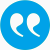#Pötzelberger, Klaus. 2014. Adaptive control variables for eestimating functionals of diffusion processes. CFE-ERCIM 2014, Pisa, Italien, 06.12.-0.8.12.. Invited Talk

BibTeX

## Abstract

The toolbox of Monte Carlo simulation provides - easy to implement - procedures for estimating functionals of stochastic processes such as the c.d.f. of marginal distributions, boundary crossing probabilities, c.d.f.'s of first exit times or prices of certain path dependent options. There exist procedures which generate paths on a discrete time grid from the exact distributions, but most procedures are biased in the sense that even on the discrete time grid, the distribution from which the samples are drawn is an approximation. The MSE (mean squared error) is then the sum of the squared bias and a variance term. If N univariate random variables are used, n discrete paths of lengths m, N=mn, are generated, the variance is of order 1/n, but the MSE is of order 1/N^\gamma with \gamma < 1. Naive applications of MC often have a MSE of order 1/\sqrt{N} only! The talk presents as variance reduction technique the method of adaptive control variables. The approximating functional is itself approximated by a functional of a discrete time path of smaller complexity. Although the expectation of the control variable has to be estimated, the combination of expectation and approximation allows an improvement of the convergence rate. Iterating the control variables leads even to a MSE which is O(1/N), the approximation rate of finite-dimensional problems. Examples of applications such as estimating boundary crossing probabilities for m-dimensional Brownian motion or diffusions and results on approximation rates are given.

## Tags

Press 'enter' for creating the tag

## Publication's profile

Status of publication Published WU Paper presented at an academic conference or symposium English Adaptive control variables for eestimating functionals of diffusion processes CFE-ERCIM 2014 2014 06.12.-0.8.12. Italy Pisa Y

## Associations

Projects
Boundary Crossing Probability
People
Pötzelberger, Klaus (Details)
Organization
Institute for Statistics and Mathematics IN (Details)